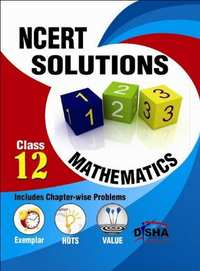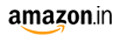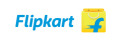# NCERT Solutions with Exemplar/HOTS/Value based Questions: Class 12 Mathematics CBSE (Arts) Class 12M.R.P 260/-192.00(26%)195.00(25%)
Product Description

The book NCERT Solutions Class 12 Mathematics is exclusively written for CBSE students of class 12. The book provides Quick Revision of the concepts involved along with Important formulas and definitions, in each chapter, which would act as a refresher.

• This is followed by the detailed solutions (Question-by-Question) of all the questions/exercises provided in the NCERT book for the current session.
• The solutions have been designed in such a manner (Step-by-Step) that it would bring 100% Concept Clarity for the student.
• The solutions are Complete (each and every question is solved), Inflow (exactly on the flow of questions in the NCERT book) and Correct (Errorless). Solutions to all In chapter Exercises and Miscellaneous exercises have been provided.
• This book is a must for all class 12 appearing students.

• Relations and Functions
• Inverse Trigonometric Functions
• Matrices
• Determinants
• Continuity and Differentiability
• Application Of Derivatives
• Integrals
• Application Of Integrals
• Differential Equation
• Vector Algebra
• Three Dimensional Geometry
• Linear Programming
• Probability